# 1. The heat transfer in a solid block can be represented by f(x, y,z)- 3x2 28y 4z2. Point M is (-2,3,5). Find the: Maxi...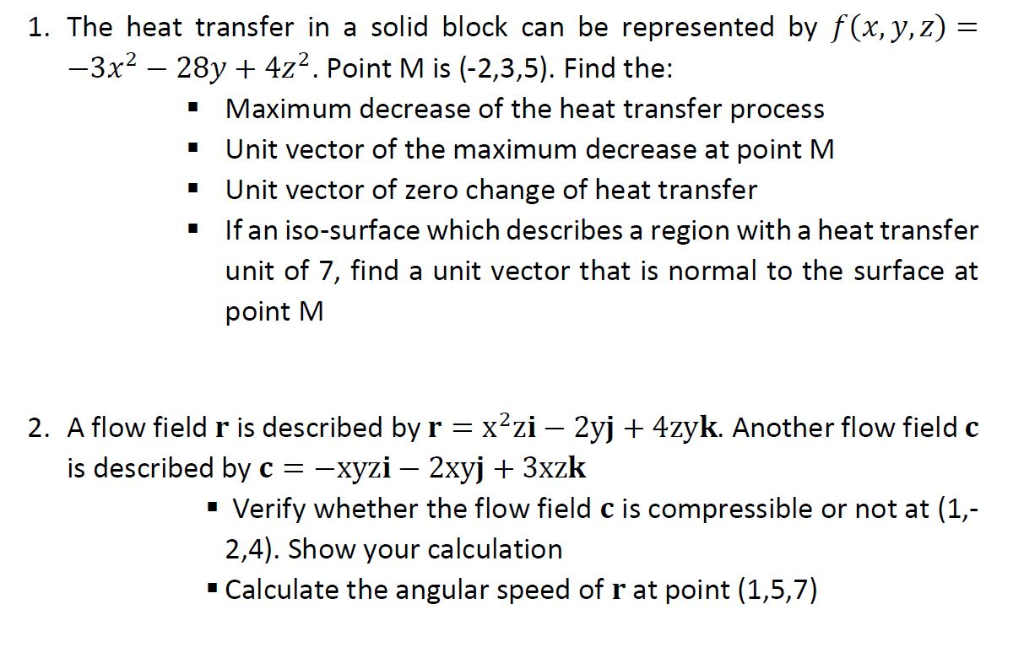1. The heat transfer in a solid block can be represented by f(x, y,z)- 3x2 28y 4z2. Point M is (-2,3,5). Find the: Maximum decrease of the heat transfer process " Unit vector of the maximum decrease at point M Unit vector of zero change of heat transfer If an iso-surface which describes a region with a heat transfer " " " unit of 7, find a unit vector that is normal to the surface at point M 2. A flow field r is described by rx2zi - 2yj + 4zyk. Another flow field c is described by c--хул-2xy + 3xzk " Verify whether the flow field c is compressible or not at (1, 2,4). Show your calculation Calculate the angular speed of r at point (1,5,7)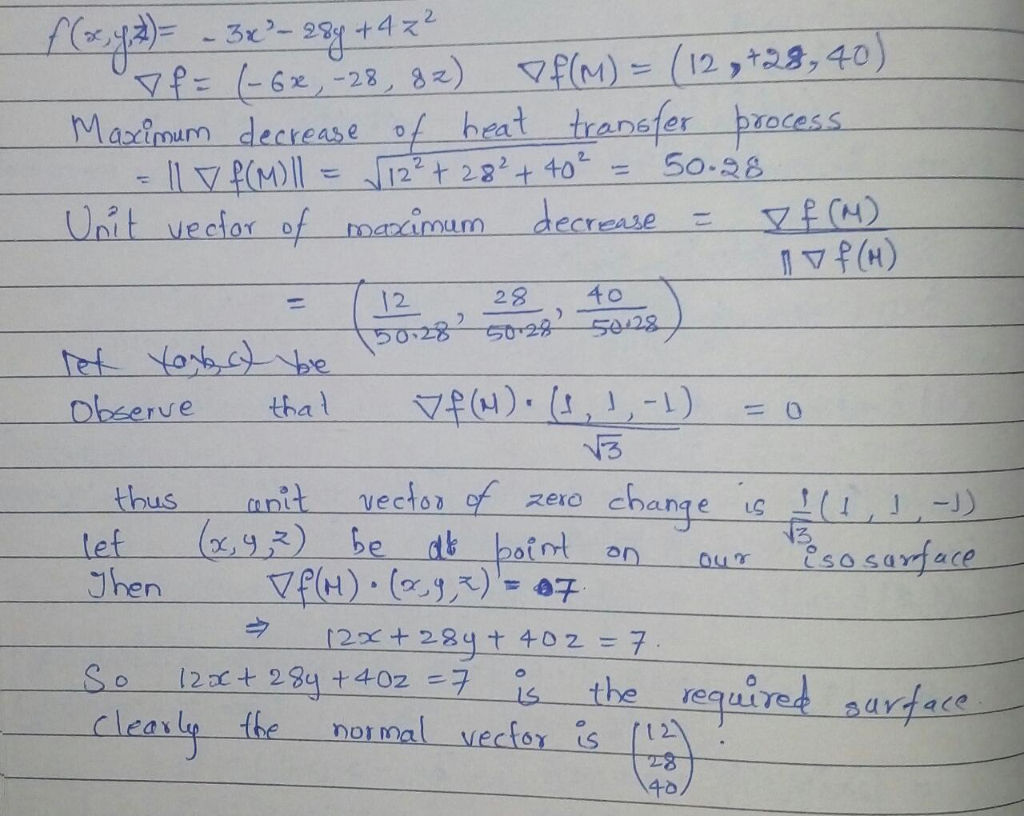first complete question according to chegg policy.

##### Add Answer of: 1. The heat transfer in a solid block can be represented by f(x, y,z)- 3x2 28y 4z2. Point M is (-2,3,5). Find the: Maxi...
Similar Homework Help Questions
• ### a) A concentration of a carbon monoxide in a tank is described by f(X,y,z) X2 + y2 + Z2. Based on Fick's Law, the d...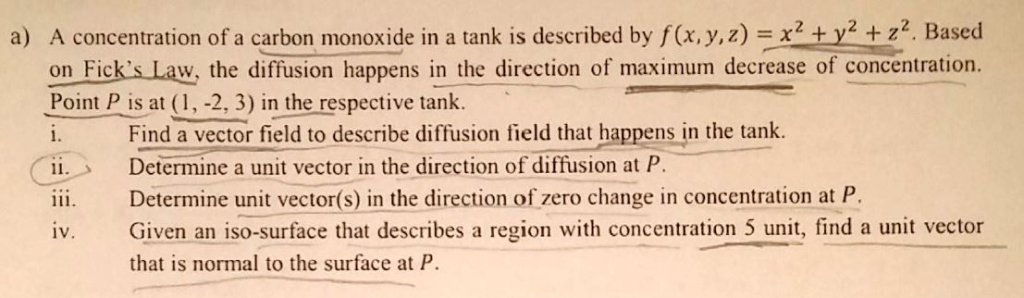a) A concentration of a carbon monoxide in a tank is described by f(X,y,z) X2 + y2 + Z2. Based on Fick's Law, the diffusion happens in the direction of maximum decrease of concentration Point P is at (1, -2, 3) in the respective tank. Find a vector field to describe diffusion field that happens in the tank. 1. Determine a unit vector in the direction of diffusion at P. ii. Determine unit vector(s) in the direction of zero change...

• ### Notation and convention: r x +y The distance from the origin to the point r [x,y,z]...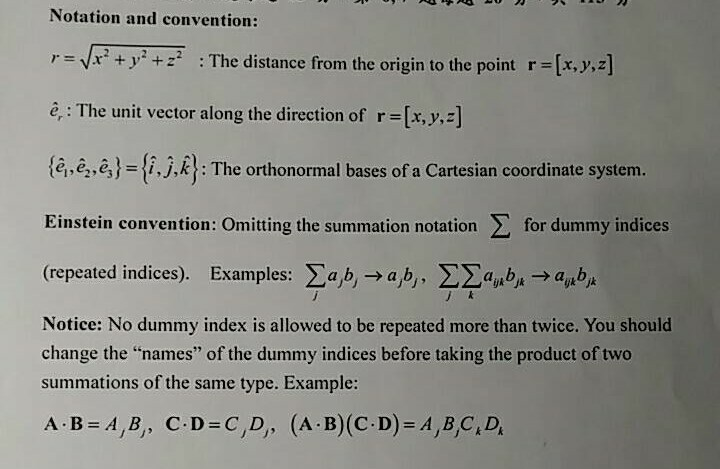Notation and convention: r x +y The distance from the origin to the point r [x,y,z] + ê: The unit vector along the direction of r-[x, y,z] (a.e,6)-i.j.):m :The orthonormal bases of a Cartesian coordinate system. for dummy indices Einstein convention: Omitting the summation notation (repeated indices). Examples:ab,-a b, ab a b Notice: No dummy index is allowed to be repeated more than twice. You should change the "names" of the dummy indices before taking the product of two summations...

• ### (1 point) This problem will illustrate the divergence theorem by computing the outward flux of the vector field F x, y, z = 2ī + 4j + k across the boundary of the right rectangular prism: 1 sx <5,...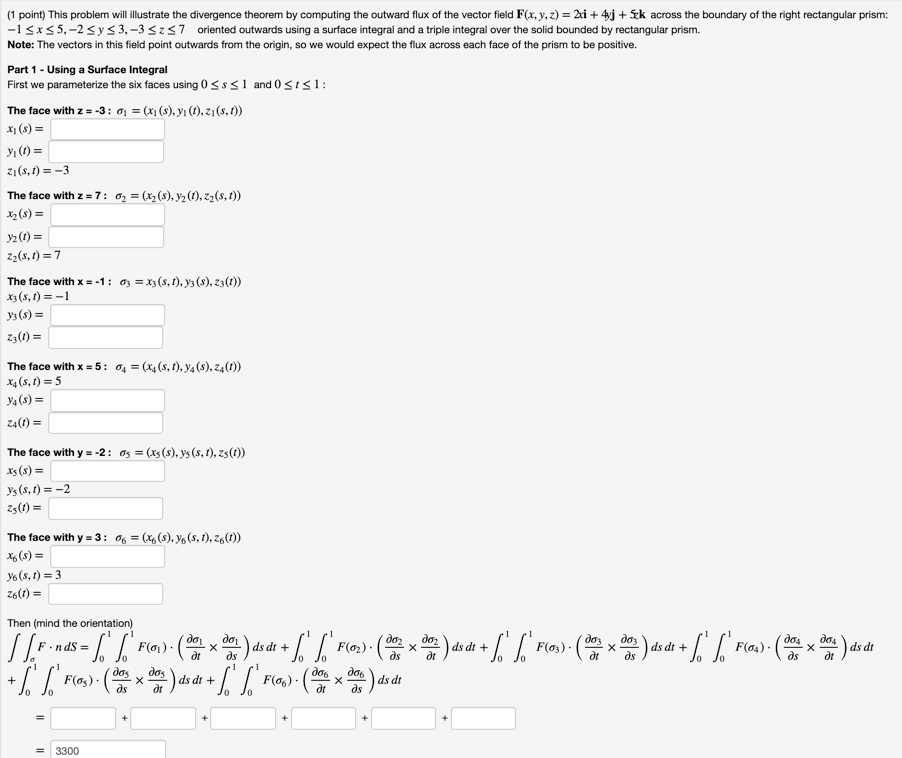(1 point) This problem will illustrate the divergence theorem by computing the outward flux of the vector field F x, y, z = 2ī + 4j + k across the boundary of the right rectangular prism: 1 sx <5,-2 Sys3,-33z37 oriented outwards using a surface integral and a triple integral over the solid bounded by rectangular prism. Note: The vectors in this field point outwards from the origin, so we would expect the flux across each face of the prism...

Need Online Homework Help?